# sklearn.preprocessing.OrdinalEncoder¶

class sklearn.preprocessing.OrdinalEncoder(*, categories='auto', dtype=<class 'numpy.float64'>, handle_unknown='error', unknown_value=None, encoded_missing_value=nan, min_frequency=None, max_categories=None)[source]

Encode categorical features as an integer array.

The input to this transformer should be an array-like of integers or strings, denoting the values taken on by categorical (discrete) features. The features are converted to ordinal integers. This results in a single column of integers (0 to n_categories - 1) per feature.

Read more in the User Guide. For a comparison of different encoders, refer to: Comparing Target Encoder with Other Encoders.

New in version 0.20.

Parameters:
categories‘auto’ or a list of array-like, default=’auto’

Categories (unique values) per feature:

• ‘auto’ : Determine categories automatically from the training data.

• list : categories[i] holds the categories expected in the ith column. The passed categories should not mix strings and numeric values, and should be sorted in case of numeric values.

The used categories can be found in the categories_ attribute.

dtypenumber type, default=np.float64

Desired dtype of output.

handle_unknown{‘error’, ‘use_encoded_value’}, default=’error’

When set to ‘error’ an error will be raised in case an unknown categorical feature is present during transform. When set to ‘use_encoded_value’, the encoded value of unknown categories will be set to the value given for the parameter unknown_value. In inverse_transform, an unknown category will be denoted as None.

New in version 0.24.

unknown_valueint or np.nan, default=None

When the parameter handle_unknown is set to ‘use_encoded_value’, this parameter is required and will set the encoded value of unknown categories. It has to be distinct from the values used to encode any of the categories in fit. If set to np.nan, the dtype parameter must be a float dtype.

New in version 0.24.

encoded_missing_valueint or np.nan, default=np.nan

Encoded value of missing categories. If set to np.nan, then the dtype parameter must be a float dtype.

New in version 1.1.

min_frequencyint or float, default=None

Specifies the minimum frequency below which a category will be considered infrequent.

• If int, categories with a smaller cardinality will be considered infrequent.

• If float, categories with a smaller cardinality than min_frequency * n_samples will be considered infrequent.

New in version 1.3: Read more in the User Guide.

max_categoriesint, default=None

Specifies an upper limit to the number of output categories for each input feature when considering infrequent categories. If there are infrequent categories, max_categories includes the category representing the infrequent categories along with the frequent categories. If None, there is no limit to the number of output features.

max_categories do not take into account missing or unknown categories. Setting unknown_value or encoded_missing_value to an integer will increase the number of unique integer codes by one each. This can result in up to max_categories + 2 integer codes.

New in version 1.3: Read more in the User Guide.

Attributes:
categories_list of arrays

The categories of each feature determined during fit (in order of the features in X and corresponding with the output of transform). This does not include categories that weren’t seen during fit.

n_features_in_int

Number of features seen during fit.

New in version 1.0.

feature_names_in_ndarray of shape (n_features_in_,)

Names of features seen during fit. Defined only when X has feature names that are all strings.

New in version 1.0.

infrequent_categories_list of ndarray

Infrequent categories for each feature.

OneHotEncoder

Performs a one-hot encoding of categorical features. This encoding is suitable for low to medium cardinality categorical variables, both in supervised and unsupervised settings.

TargetEncoder

Encodes categorical features using supervised signal in a classification or regression pipeline. This encoding is typically suitable for high cardinality categorical variables.

LabelEncoder

Encodes target labels with values between 0 and n_classes-1.

Notes

With a high proportion of nan values, inferring categories becomes slow with Python versions before 3.10. The handling of nan values was improved from Python 3.10 onwards, (c.f. bpo-43475).

Examples

Given a dataset with two features, we let the encoder find the unique values per feature and transform the data to an ordinal encoding.

>>> from sklearn.preprocessing import OrdinalEncoder
>>> enc = OrdinalEncoder()
>>> X = [['Male', 1], ['Female', 3], ['Female', 2]]
>>> enc.fit(X)
OrdinalEncoder()
>>> enc.categories_
[array(['Female', 'Male'], dtype=object), array([1, 2, 3], dtype=object)]
>>> enc.transform([['Female', 3], ['Male', 1]])
array([[0., 2.],
[1., 0.]])

>>> enc.inverse_transform([[1, 0], [0, 1]])
array([['Male', 1],
['Female', 2]], dtype=object)


By default, OrdinalEncoder is lenient towards missing values by propagating them.

>>> import numpy as np
>>> X = [['Male', 1], ['Female', 3], ['Female', np.nan]]
>>> enc.fit_transform(X)
array([[ 1.,  0.],
[ 0.,  1.],
[ 0., nan]])


You can use the parameter encoded_missing_value to encode missing values.

>>> enc.set_params(encoded_missing_value=-1).fit_transform(X)
array([[ 1.,  0.],
[ 0.,  1.],
[ 0., -1.]])


Infrequent categories are enabled by setting max_categories or min_frequency. In the following example, “a” and “d” are considered infrequent and grouped together into a single category, “b” and “c” are their own categories, unknown values are encoded as 3 and missing values are encoded as 4.

>>> X_train = np.array(
...     [["a"] * 5 + ["b"] * 20 + ["c"] * 10 + ["d"] * 3 + [np.nan]],
...     dtype=object).T
>>> enc = OrdinalEncoder(
...     handle_unknown="use_encoded_value", unknown_value=3,
...     max_categories=3, encoded_missing_value=4)
>>> _ = enc.fit(X_train)
>>> X_test = np.array([["a"], ["b"], ["c"], ["d"], ["e"], [np.nan]], dtype=object)
>>> enc.transform(X_test)
array([[2.],
[0.],
[1.],
[2.],
[3.],
[4.]])


Methods

 fit(X[, y]) Fit the OrdinalEncoder to X. fit_transform(X[, y]) Fit to data, then transform it. get_feature_names_out([input_features]) Get output feature names for transformation. Get metadata routing of this object. get_params([deep]) Get parameters for this estimator. Convert the data back to the original representation. set_output(*[, transform]) Set output container. set_params(**params) Set the parameters of this estimator. Transform X to ordinal codes.
fit(X, y=None)[source]

Fit the OrdinalEncoder to X.

Parameters:
Xarray-like of shape (n_samples, n_features)

The data to determine the categories of each feature.

yNone

Ignored. This parameter exists only for compatibility with Pipeline.

Returns:
selfobject

Fitted encoder.

fit_transform(X, y=None, **fit_params)[source]

Fit to data, then transform it.

Fits transformer to X and y with optional parameters fit_params and returns a transformed version of X.

Parameters:
Xarray-like of shape (n_samples, n_features)

Input samples.

yarray-like of shape (n_samples,) or (n_samples, n_outputs), default=None

Target values (None for unsupervised transformations).

**fit_paramsdict

Returns:
X_newndarray array of shape (n_samples, n_features_new)

Transformed array.

get_feature_names_out(input_features=None)[source]

Get output feature names for transformation.

Parameters:
input_featuresarray-like of str or None, default=None

Input features.

• If input_features is None, then feature_names_in_ is used as feature names in. If feature_names_in_ is not defined, then the following input feature names are generated: ["x0", "x1", ..., "x(n_features_in_ - 1)"].

• If input_features is an array-like, then input_features must match feature_names_in_ if feature_names_in_ is defined.

Returns:
feature_names_outndarray of str objects

Same as input features.

Get metadata routing of this object.

Please check User Guide on how the routing mechanism works.

Returns:

A MetadataRequest encapsulating routing information.

get_params(deep=True)[source]

Get parameters for this estimator.

Parameters:
deepbool, default=True

If True, will return the parameters for this estimator and contained subobjects that are estimators.

Returns:
paramsdict

Parameter names mapped to their values.

property infrequent_categories_

Infrequent categories for each feature.

inverse_transform(X)[source]

Convert the data back to the original representation.

Parameters:
Xarray-like of shape (n_samples, n_encoded_features)

The transformed data.

Returns:
X_trndarray of shape (n_samples, n_features)

Inverse transformed array.

set_output(*, transform=None)[source]

Set output container.

See Introducing the set_output API for an example on how to use the API.

Parameters:
transform{“default”, “pandas”}, default=None

Configure output of transform and fit_transform.

• "default": Default output format of a transformer

• "pandas": DataFrame output

• None: Transform configuration is unchanged

Returns:
selfestimator instance

Estimator instance.

set_params(**params)[source]

Set the parameters of this estimator.

The method works on simple estimators as well as on nested objects (such as Pipeline). The latter have parameters of the form <component>__<parameter> so that it’s possible to update each component of a nested object.

Parameters:
**paramsdict

Estimator parameters.

Returns:
selfestimator instance

Estimator instance.

transform(X)[source]

Transform X to ordinal codes.

Parameters:
Xarray-like of shape (n_samples, n_features)

The data to encode.

Returns:
X_outndarray of shape (n_samples, n_features)

Transformed input.

## Examples using sklearn.preprocessing.OrdinalEncoder¶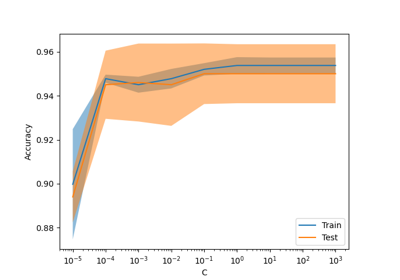Release Highlights for scikit-learn 1.3

Release Highlights for scikit-learn 1.3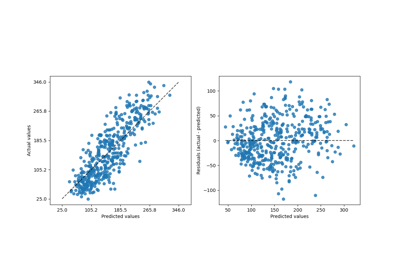Release Highlights for scikit-learn 1.2

Release Highlights for scikit-learn 1.2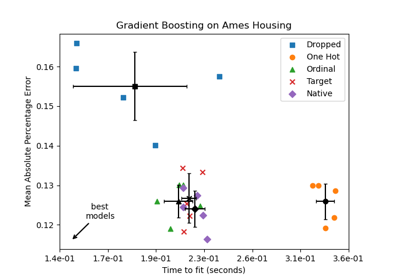Categorical Feature Support in Gradient Boosting

Categorical Feature Support in Gradient Boosting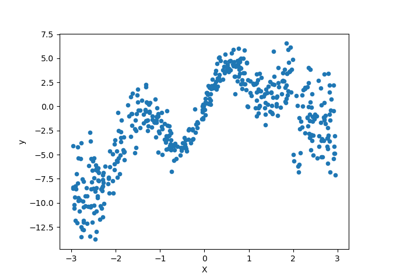Combine predictors using stacking

Combine predictors using stacking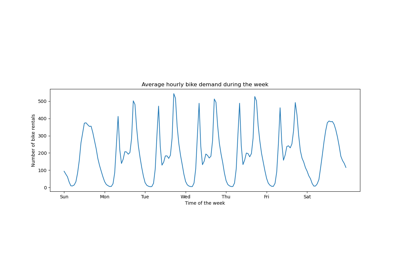Time-related feature engineering

Time-related feature engineering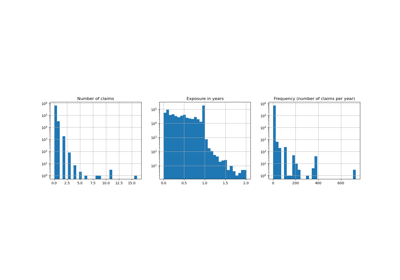Poisson regression and non-normal loss

Poisson regression and non-normal loss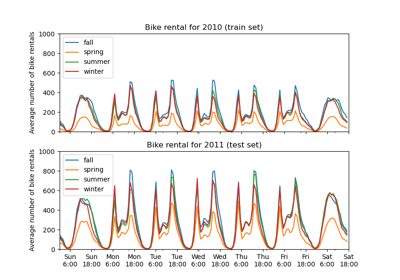Partial Dependence and Individual Conditional Expectation Plots

Partial Dependence and Individual Conditional Expectation Plots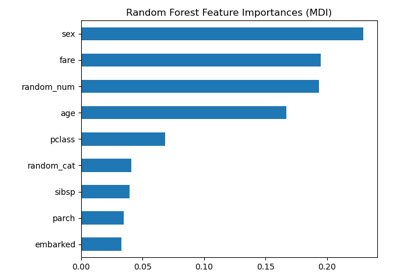Permutation Importance vs Random Forest Feature Importance (MDI)

Permutation Importance vs Random Forest Feature Importance (MDI)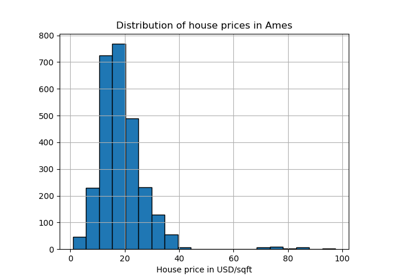Evaluation of outlier detection estimators

Evaluation of outlier detection estimators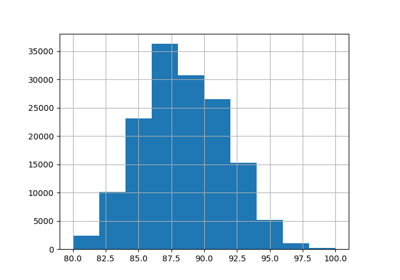Comparing Target Encoder with Other Encoders

Comparing Target Encoder with Other Encoders